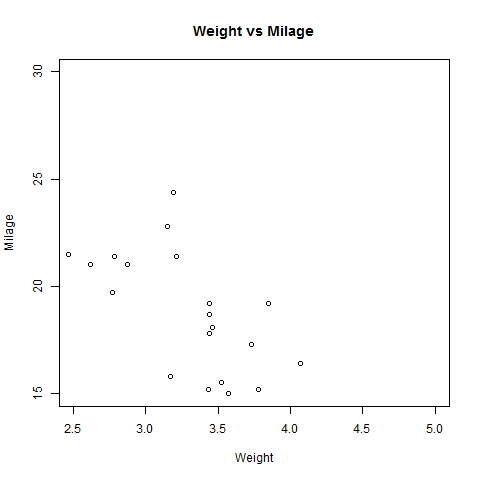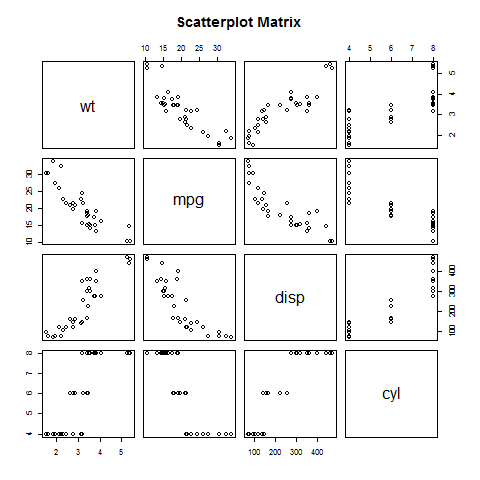# R语言 散点图

2022-06-16 15:42 更新

## 语法

```plot(x, y, main, xlab, ylab, xlim, ylim, axes)
```

• x是其值为水平坐标的数据集。

• y是其值是垂直坐标的数据集。

• main要是图形的图块。

• xlab是水平轴上的标签。

• ylab是垂直轴上的标签。

• xlim是用于绘图的x的值的极限。

• ylim是用于绘图的y的值的极限。

• axes指示是否应在绘图上绘制两个轴。

## 例

```input <- mtcars[,c('wt','mpg')]
```

```                    wt      mpg
Mazda RX4           2.620   21.0
Mazda RX4 Wag       2.875   21.0
Datsun 710          2.320   22.8
Hornet 4 Drive      3.215   21.4
Valiant             3.460   18.1
```

## 创建散点图

```# Get the input values.
input <- mtcars[,c('wt','mpg')]

# Give the chart file a name.
png(file = "scatterplot.png")

# Plot the chart for cars with weight between 2.5 to 5 and mileage between 15 and 30.
plot(x = input\$wt,y = input\$mpg,
xlab = "Weight",
ylab = "Milage",
xlim = c(2.5,5),
ylim = c(15,30),
main = "Weight vs Milage"
)

# Save the file.
dev.off()
```## 散点图矩阵

### 语法

```pairs(formula, data)
```

• formula表示成对使用的一系列变量。

• data表示将从其获取变量的数据集。

### 例

```# Give the chart file a name.
png(file = "scatterplot_matrices.png")

# Plot the matrices between 4 variables giving 12 plots.

# One variable with 3 others and total 4 variables.

pairs(~wt+mpg+disp+cyl,data = mtcars,
main = "Scatterplot Matrix")

# Save the file.
dev.off()
```App下载